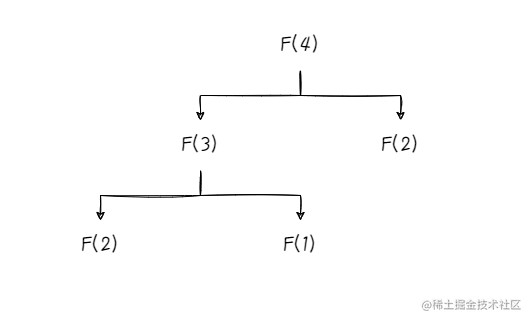# 04.编程技巧：从斐波那契数列到递归## 斐波那契数列

$F(4)=F(3)+F(2)=(F(2)+F(1))+F(1)$public int fib(int n) {
if (n < 3) {
return 1;
}
return fib(n - 1) + fib(n - 2);
}

## 递归

### 尾递归

long factorial(long n, long result) {
if (n == 0) {
return 0;
} else if (n == 1) {
return result;
}
return factorial(n - 1, result * n);
}

### 尾调用消除

• 调用栈使用栈这种结构，特点是先进后出；
• 调用栈使用栈帧存储方法的执行信息。

## 复杂度计算

### 递归跟踪（递归树）

$2^OT(n)+2^1T(n)+2^2T(n)+(2^3-2)T(n)+(2^4-14)T(n)\approx2^{n-2}T(n)$

$\Theta(n)=\frac{(1+\sqrt{5})^n}{2}\approx1.618^n$